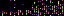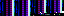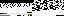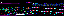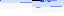```from random import randint
from operator import sub

from pygame import Surface

class Barrier(GameChild, Surface):

dir_r, dir_l = 1, -1

def __init__(self, parent, y, sibling=None):
GameChild.__init__(self, parent)
self.y = y
self.sibling = sibling
self.init_surface()

def init_surface(self):
size = self.determine_size()
Surface.__init__(self, (size, size))
self.fill(self.parent.transparent_color)
self.set_rect()

def determine_size(self):
parent = self.parent
size_range = parent.size_range
pos = float(self.y) / -sub(*parent.y_range)
return pos * -sub(*size_range) + size_range

def set_rect(self):
rect = self.get_rect()
rect.top = self.y
sibling = self.sibling
if not sibling:
growth = self.dir_r
rect.top = self.y
rect.left = self.generate_initial_x()
else:
growth = sibling.growth
if growth == self.dir_r:
rect.left = sibling.rect.right
if rect.right > self.parent.get_width():
growth = self.dir_l
rect.right = sibling.rect.left
if growth == self.dir_l:
rect.right = sibling.rect.left
if rect.left < 0:
growth = self.dir_r
rect.left = sibling.rect.right
self.growth = growth
self.rect = rect
self.x = rect.left
self.set_step()

def generate_initial_x(self):
parent = self.parent
return randint(0, parent.get_width() - parent.size_range)

if self.rect.centerx > self.parent.get_width() / 2:
else:

def set_step(self):
parent = self.parent
center = parent.get_width() / 2
rnge = abs((self.rect.centerx - center) * 2)
self.step = float(rnge) / parent.step_limit

else:

def update(self):
self.move()
self.draw()

def move(self):
self.rect.left = self.x

def draw(self):
self.parent.area.blit(self, self.rect)
```
```from esp_hadouken.levels.Level import *
from Gauntlet import *

class Horse(Level):

def __init__(self, parent):
Level.__init__(self, parent)

def set_void(self):
self.void = Gauntlet(self)
```
```from random import randint
from operator import sub

from pygame import draw

class Bubble(GameChild):

def __init__(self, parent, y=None):
GameChild.__init__(self, parent)
self.y = y
self.set_x()

def set_x(self):
self.x = self.parent.get_width() / 2

def update(self):
self.y += self.parent.scroll_speed

parent = self.parent
pos = float(self.y) / -sub(*parent.y_range)

def draw(self):
parent = self.parent
center = self.x, self.y
```
```from random import randint

from pygame import Color, Surface

from Bubble import *

class Void(levels.Void.Void):

def __init__(self, parent):
levels.Void.Void.__init__(self, parent)
self.iterations = 0
self.set_area()
self.show()

config = self.get_configuration()
self.switch_frequency = config["tooth-level-switch-frequency"]
self.scroll_speed = config["tooth-level-scroll-speed"]
self.spawn_range = config["tooth-level-spawn-range"]

def set_area(self):
self.set_y_range()
y_range = self.y_range
height = y_range - y_range
area = Surface((self.parent.get_width(), height)).convert()
self.area_bg = Surface(area.get_size()).convert()
self.area = area
self.generate_bubbles()

def set_y_range(self):
parent = self.parent
self.y_range = start, end

def generate_bubbles(self):
self.bubbles = []
y = self.get_height()

if y is None:
self.bubbles.insert(0, Bubble(self, y))
self.next_spawn = randint(*self.spawn_range)

def toggle(self):
if self.visible:
self.hide()
else:
self.show()

def show(self):
self.visible = True

def hide(self):
self.visible = False

def update_area(self):
self.update_bubbles()
iterations = self.iterations + 1
if 1.0 / iterations <= self.switch_frequency:
self.toggle()
iterations = 0
if self.visible:
self.clear_area()
self.draw_area()
self.iterations = iterations

def update_bubbles(self):
bubbles = self.bubbles
if bubbles.y - radius_range > self.next_spawn:
for bubble in bubbles:
if bubble.y > self.y_range + radius_range:
bubbles.remove(bubble)
else:
bubble.update()

def clear_area(self):
self.area.blit(self.area_bg, (0, 0))

def draw_area(self):
self.draw_bubbles()
self.blit(self.area, (0, self.y_range))

def draw_bubbles(self):
for bubble in self.bubbles:
bubble.draw()
```
44.210.21.70
44.210.21.70
44.210.21.70

September 13, 2013

```from array import array
from time import sleep

import pygame
from pygame.mixer import Sound, get_init, pre_init

class Note(Sound):

def __init__(self, frequency, volume=.1):
self.frequency = frequency
Sound.__init__(self, self.build_samples())
self.set_volume(volume)

def build_samples(self):
period = int(round(get_init() / self.frequency))
samples = array("h",  * period)
amplitude = 2 ** (abs(get_init()) - 1) - 1
for time in xrange(period):
if time < period / 2:
samples[time] = amplitude
else:
samples[time] = -amplitude
return samples

if __name__ == "__main__":
pre_init(44100, -16, 1, 1024)
pygame.init()
Note(440).play(-1)
sleep(5)
```

This program generates and plays a 440 Hz tone for 5 seconds. It can be extended to generate the spectrum of notes with a frequency table or the frequency formula. Because the rewards in Send are idealized ocean waves, they can also be represented as tones. Each level has a tone in its goal and a tone based on where the player's disc lands. Both play at the end of a level, sounding harmonic for a close shot and discordant for a near miss. The game can dynamically create these tones using the program as a basis.

I'm also building an algorithmically generated song: Silk Routes (Scissored). Here is an example of how it sounds so far.